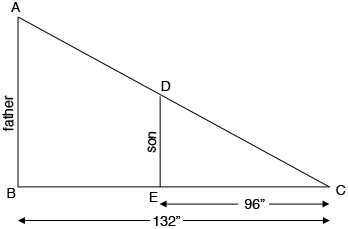SEARCH HOMEMath Central Quandaries & QueriesQuestion from esada, a student: the father and son cast a shadow of 11 feet and 8 feet,respectively if the son is 4'8'' in height,how tall is the father?I converted the lengths of the shadows to inches. I then had the son stand in his father's shadow so that the tips of their shadows were at the same point $C.$Since the father and son are both standing perpendicular to the ground it is clear that $ABC$ and $DEC$ are similar triangles. You also know that the son is 4'8" = 56" tall.

Can you complete the problem now?

PennyMath Central is supported by the University of Regina and The Pacific Institute for the Mathematical Sciences.